# 二项分布

 "二项" 在这里的意思是关于有"二"个 …… …… 结果或部分的事物（像自行车）。抛硬币： 结果是正面（H），还是 反面（T） 我们说：硬币正面向上（H）的概率是 ½ 反面向上（T）的概率是 ½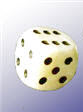掷骰子： 结果是四 …… …… 吗？ 我们说：四 的概率是 1/6 （六面里有一面是四）。 不是四的概率是 5/6 （六面里有五面不是四）

## 来抛硬币！

 HHHHHTHTHHTTTHHTHTTTHTTT### 我们想要的是哪些结果？

"两个正面" 可以是任何次序： "HHT"、"THH" 和 "HTH" 都有两个正面（和一个反面）。

### 每个结果的概率是多少？

 需要的 结果 每个结果的 概率 3 × 1/8 =  3/8

• 结果：抛三次硬币的结果（8 个可能）
• 事件： 三次里有"两个正面" （3个可能）

## 我们来计算所有的概率：

• P(三个正面) = P(HHH) = 1/8
• P(两个正面) = P(HHT) + P(HTH) + P(THH) = 1/8 + 1/8 + 1/8 = 3/8
• P(一个正面) = P(HTT) + P(THT) + P(TTH) = 1/8 + 1/8 + 1/8 = 3/8
• P(没有正面) = P(TTT) = 1/8

• P(X = 3) = 1/8
• P(X = 2) = 3/8
• P(X = 1) = 3/8
• P(X = 0) = 1/8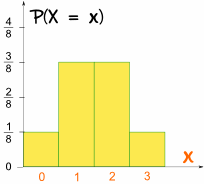## 做个公式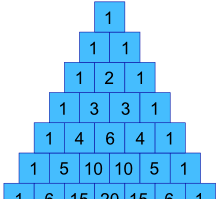这些值是 杨辉三角 的第三行 …… ！   我们可以用一个公式来计算这些值吗？
 可以的，像这样：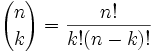n = 总数 k = 我们想求的数 通常这个是叫 "n取k"。你可去 组合与排列 来了解更多。 注意： "!" 的意思是 "阶乘"，例如 4! = 1×2×3×4 = 24

### 例子：抛 3次，结果有 2个正面

n=3k=2

 n! = 3! = 3×2×1 = 3 k!(n-k)! 2!(3-2)! 2×1 × 1

（在上面我们数出来了，不过这里是用公式算出来的。）

### 例子：抛 9次，结果有 5个正面的可能性是多少？

n=9k=5

 n! = 9! = 9×8×7×6×5×4×3×2×1 = 126 k!(n-k)! 5!(9-5)! 5×4×3×2×1 × 4×3×2×1

 我们想要的 结果的次数 每个结果的 概率 126 × 1 = 126 512 512

 P(X=5)  = 126 = 63 = 0.24609375 512 256

（比全部写下来容易点。）

## 偏差！

### 接下来的三个顾客买两个鸡肉三明治的概率是多少？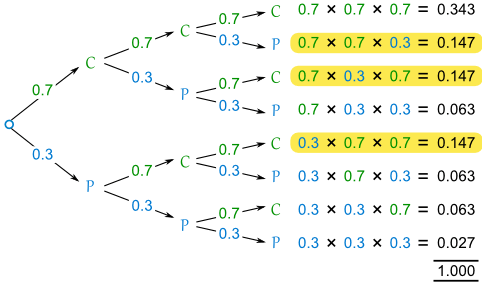• 0.7 是我们想要的结果的概率，称它为 p
• 2 是我们想要的结果的个数，称它为 k

• 其他的结果的概率是：1-p
• 一共有 n 个结果
• 其他的结果的个数是：n-k
"其他的结果" （一个猪肉）的概率是：(1-p)(n-k)

pk(1-p)(n-k)

### 例子：（续）

• p = 0.7 （鸡肉的概率）
• n = 3
• k = 2

pk(1-p)(n-k) = 0.72(1-0.7)(3-2) = 0.72(0.3)(1) = 0.7 × 0.7 × 0.3 = 0.147

 n! = 3! = 3×2×1 = 3 k!(n-k)! 2!(3-2)! 2×1 × 1

 我们想要的 结果 每个结果的 概率 3 × 0.147 = 0.441

### 例子："因为 70% 的人选鸡肉，所以下 10个顾客里有 7个会买鸡肉" …… 这句话是对的可能性是多少？

• p = 0.7
• n = 10
• k = 7

pk(1-p)(n-k) = 0.77(1-0.7)(10-7) = 0.77(0.3)(3) = 0.0022235661

 n! = 10! k!(n-k)! 7!(10-7)!
 = 10×9×8×7×6×5×4×3×2×1 7×6×5×4×3×2×1 × 3×2×1
 = 10×9×8 = 120 3×2×1

 我们想要的 结果 每个结果的 概率 120 × 0.0022235661 = 0.266827932

## 结合起来

 n! k!(n-k)!

pk(1-p)(n-k)

n个里有 k个的概率：

 P(n取k) = n! pk(1-p)(n-k) k!(n-k)!

• 试验是独立的，
• 每个试验只有两个可能结果，
• 每个试验里的 "成功" 概率是不变的。

## 梅花机## 掷骰子

• 0 个二点
• 1 个二点
• 2 个二点
• 3 个二点
• 4 个二点

n=4, p = P(二点) = 1/6

X 是这个随机变量："掷四次骰子里得到二点的次数"。

 P(n取k) = n! pk(1-p)(n-k) k!(n-k)!

• P(X = 0) = (4!/0!4!) × (1/6)0(5/6)4 = 1 × 1 × (5/6)4 = 0.4823
• P(X = 1) = (4!/1!3!) × (1/6)1(5/6)3 = 4 × (1/6) × (5/6)3 = 0.3858
• P(X = 2) = (4!/2!2!) × (1/6)2(5/6)2 = 6 × (1/6)2 × (5/6)2 = 0.1157
• P(X = 3) = (4!/3!1!) × (1/6)3(5/6)1 = 4 × (1/6)3 × (5/6) = 0.0154
• P(X = 4) = (4!/4!0!) × (1/6)4(5/6)0 = 1 × (1/6)4 × 1 = 0.0008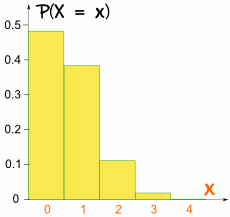## 运动自行车

• n = 4,
• p = P(通过) = 0.9

X 是代表 "在四个质量检查里通过的个数" 的随机变量

 P(n取k) = n! pk(1-p)(n-k) k!(n-k)!

• P(X = 0) = (4!/0!4!) × 0.900.14 = 1 × 1 × 0.0001 = 0.0001
• P(X = 1) = (4!/1!3!) × 0.910.13 = 4 × 0.9 × 0.001 = 0.0036
• P(X = 2) = (4!/2!2!) × 0.920.12 = 6 × 0.81 × 0.01 = 0.0486
• P(X = 3) = (4!/3!1!) × 0.930.11 = 4 × 0.729 × 0.1 = 0.2916
• P(X = 4) = (4!/4!0!) × 0.940.10 = 1 × 0.6561 × 1 = 0.6561

## 平均、方差和标准差

μ = np

μ = 4 × 0.9 = 3.6

σ = √(np(1-p))

σ = √(0.36) = 0.6

 X P(X) X × P(X) X2 × P(X) 0 0.0001 0 0 1 0.0036 0.0036 0.0036 2 0.0486 0.0972 0.1944 3 0.2916 0.8748 2.6244 4 0.6561 2.6244 10.4976 总和： 3.6 13.32

μ = 3.6

σ = √(0.36) = 0.6

## 总结

 P(n取k) = n! pk(1-p)(n-k) k!(n-k)!

X 的平均值：μ = np

X 的方差：σ2 = np(1-p)
X 的标准差：σ = √(np(1-p))